Scroll

# Programming Col Sig (Correlations): Classical Student Test

Follow

This article demonstrates how to script the Student Test formula for two different Correlation outputs: First, the significance test of a correlation between two variables (with N respondents in common). Second, the significance test of a correlation between two variables (in two independent populations).

The .qes file and portfolio containing the examples discussed can be downloaded here.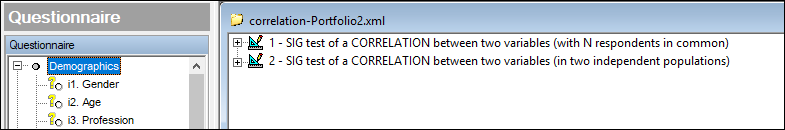Sig test of a correlation between two variables (with N respondents in common)

The formula is: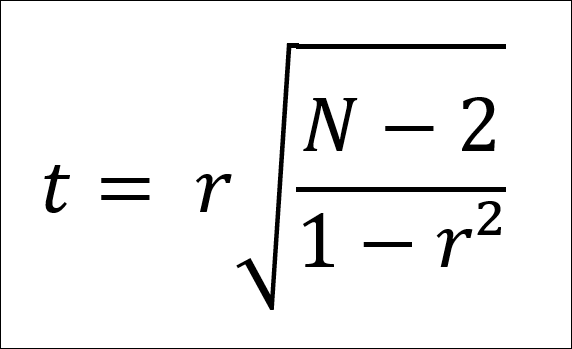To test whether a correlation r between 2 variables with N respondents in common is significantly different from zero.

t is the sigma value

• If t > 1.645, significance at 90%
• If t > 1.960, significance at 95%
• If t > 2.576, significance at 99%

The test follows a reduced normal centred law on which a Student's test can be applied.

An example correlation table definition is shown below: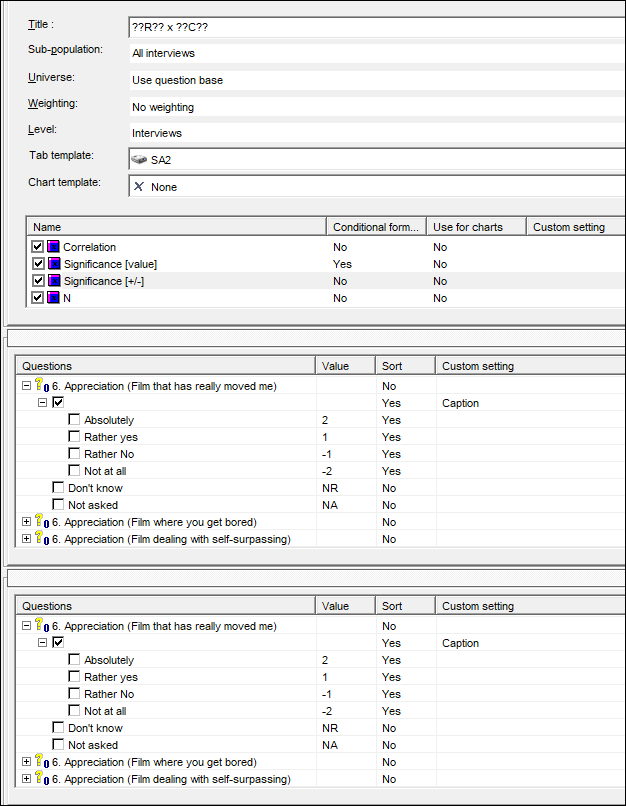The result: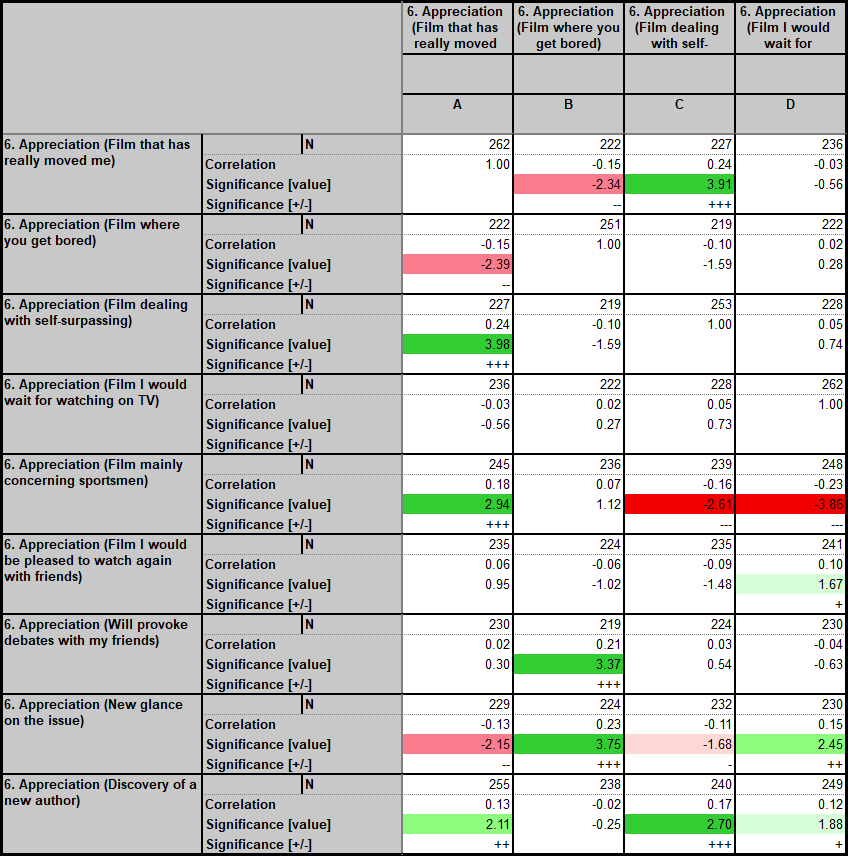The Significance [value] calculation is done using calculation arithmetic:

```Dim r1 = CurrentTable.GetCell(CurrentCell.X,CurrentCell.Y-1).Value
Dim n1 = CurrentCell.Row.Question.Data.FilterbyXY().Size()

Dim Denom1 = 1 - Pow(r1,2)
Dim tValue = r1 * (Pow( ( (n1-2) / Denom1 ) , 0.5))

If r1 <> 1 Then
Return tValue
Else
Return nr
Endif
```

The conditional formatting is set on this calculation as follows: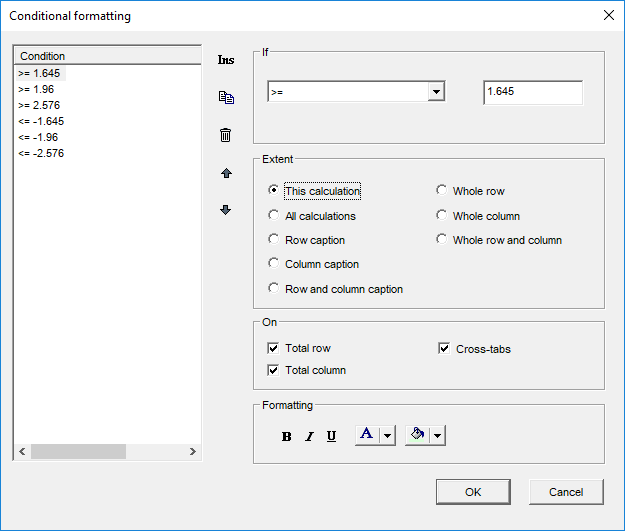The Significance [+/-] calculation can then be done using calculation arithmetic:

```Dim r1 = CurrentTable.GetCell(CurrentCell.X,CurrentCell.Y-2).Value
Dim s1 = CurrentTable.GetCell(CurrentCell.X,CurrentCell.Y-1).Value
'+++
If ((s1 >= 1.645 and s1 < 1.96)) and r1<>1 Then
Return "+"
Endif
If ((s1 >= 1.96 and s1 < 2.576)) and r1<>1 Then
Return "++"
Endif
If (s1 >= 2.576) and r1<>1 Then
Return "+++"
Endif
'---
If ((s1 <= -1.645 and s1 > -1.96)) and r1<>1 Then
Return "-"
Endif
If ((s1 <= -1.96 and s1 > -2.576)) and r1<>1 Then
Return "--"
Endif
If (s1 <= -2.576) and r1<>1 Then
Return "---"
Endif```

If you just want the colouring to show the significance you can hide both these calculations and set the conditional formatting to take effect on 'All calculations'.

Sig test of a correlation between two variables (in two independent populations)

The formula is: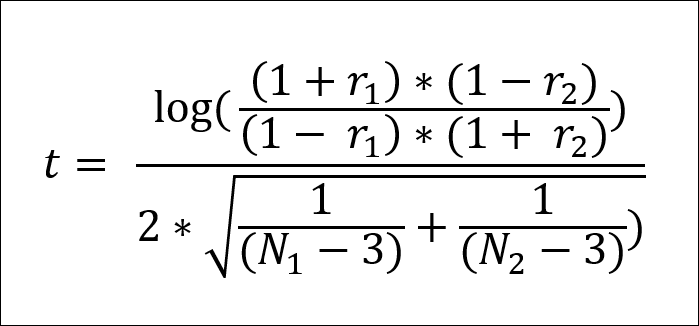Where r1 is the correlation between 2 variables on a sample size of N1 and r2 is the correlation between the same 2 variables on a second independent sample size of N2

again t is the sigma value

• If t > 1.645, significance at 90%
• If t > 1.960, significance at 95%
• If t > 2.576, significance at 99%

The test follows a reduced normal centred law on which a Student's test can be applied.

The table set-up is similar with the inbuilt Correlation and N (count) calculations used. However, in order to provide the independent sample columns we put the variable, i2. Age, in the edges and select the option break option shown below: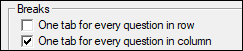The result: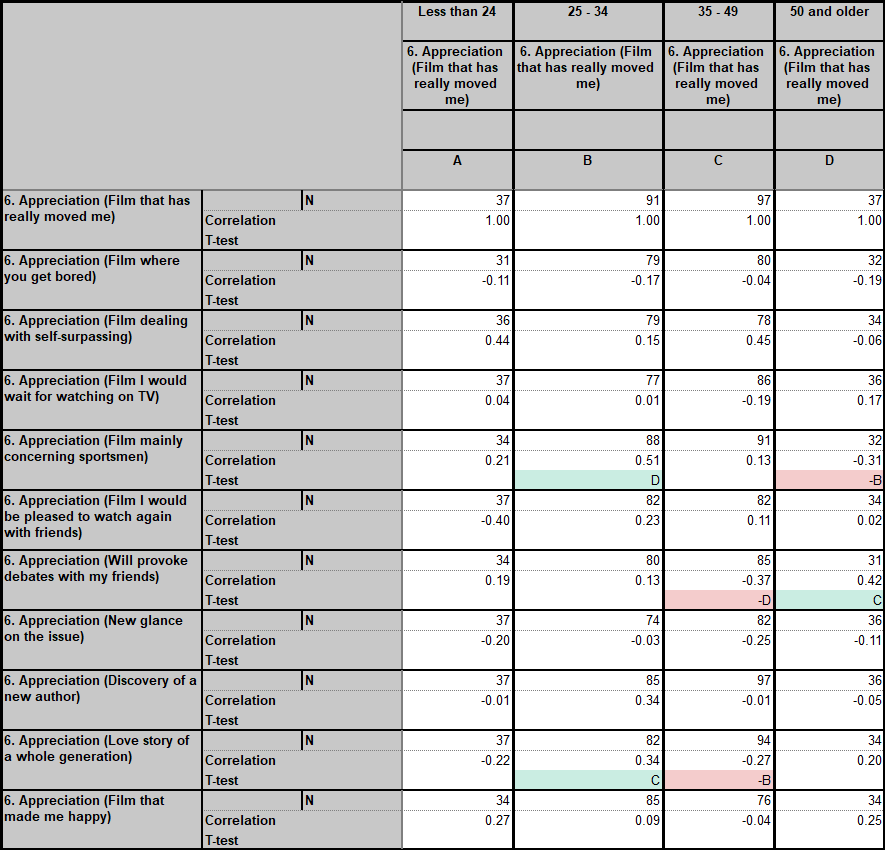The calculation which returns the col sig letters is T-Test.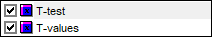The other calculation, T-values, returns the sigma values of the current column against all the others in an array. This can be useful for checking e.g.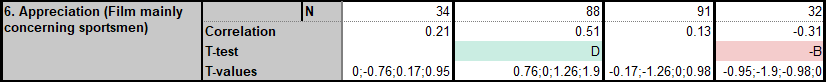The calculation arithmetic script for T-Test is:

```Dim N1 = CurrentCell.Row.Question.Data.FilterByXY().Size()
Dim R1 = CurrentTable.GetCell(CurrentCell.X,CurrentCell.Y-1).Value

Dim strColsig = ""
Dim i = 0

For i = currentTable.StartX to currentTable.MaxX

Dim N2 = CurrentCell.Row.Question.Data.FilterByXY(i).Size()
Dim R2 = CurrentTable.GetCell(i,CurrentCell.Y-1).Value

Dim dDenomP = ( ( (1+R1) * (1-R2) ) / ( (1-R1) * (1+R2) ) )
Dim dNumeratorP = ( ( 1 / (N1-3) ) + ( 1 / (N2-3) ) )
Dim dSigma = dDenomP.Log10() / ( 2 * ( dNumeratorP.Pow(0.5) ) )' The test is done at 90% and the letter is shown with a minus sign if significantly lower
If dSigma.Abs() > 1.65 and R1 > R2 Then
strColsig = strColsig + CurrentTable.GetColSigLetter(i,2)
ElseIf dSigma.Abs() > 1.65 and R2 > R1 Then
strColsig = strColsig + CurrentTable.GetColSigLetter(i,-2)
Endif

Next i
Return strColsig```

The conditional formatting this time is taken care of by a cleaning script (General tab > Settings > Sorting).

```' Conditional formatting
Dim i = 0
Dim j = 0
Dim k = 0
Dim h = 0

Dim arrColSigLetters = {""}
For i = currentTable.StartX to currentTable.MaxX
arrColSigLetters = arrColSigLetters + CurrentTable.GetColSigLetter(i)
Next i

arrColSigLetters = arrColSigLetters.RemoveAt(1)

For k = currentTable.StartX to currentTable.MaxX
For j = currentTable.StartY to currentTable.MaxY
For h = 1 to arrColSigLetters.Count
If Instr((arrColSigLetters[h]).ToString(), CurrentTable.GetCell(k,j).Text) and Instr("-", CurrentTable.GetCell(k,j).Text) Then
CurrentTable.GetCell(k,j).SetBackColor(244,204,204)
ElseIf Instr((arrColSigLetters[h]).ToString(), CurrentTable.GetCell(k,j).Text) Then
CurrentTable.GetCell(k,j).SetBackColor(202,237,226)
EndIf
Next h
Next j
Next k
```